# 2.1 Formulas for Symmetries in Cartesian Coordinates

In the formulas below, a multiplication between a matrix and a pair of coordinates should be carried out regarding the pair as a column vector (or a matrix with two rows and one column). Thus(x,y)=(ax+by, cx+dy).

Translation by (x,y):

(x,y)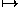(x+x, y+y)

Rotation through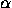(counterclockwise) around the origin: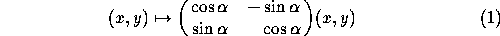Rotation through(counterclockwise) around an arbitrary point (x,y):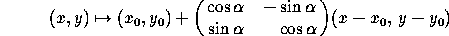Reflection in the x-axis:

(x,y)(x,-y)

Reflection in the y-axis:

(x,y)(-x,y)

Reflection in the xy-diagonal:

(x,y)(y,x)

Reflection in a line with equation ax+by+c=0: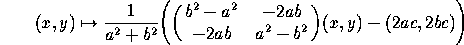Reflection in a line going through (x,y) and making an anglewith the x-axis: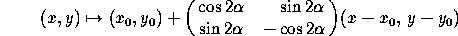Glide-reflection in a line L with displacement d: Apply first a reflection in L, then a translation by a vector of length d in the direction of L, that is, by the vectorif L has equation ax+by+c=0.

Next: 2.2 Formulas for Symmetries in Homogeneous Coordinates
Up: 2 Plane Symmetries or Isometries
Previous: 2 Plane Symmetries or IsometriesThe Geometry Center Home Page

Silvio Levy
Wed Oct 4 16:41:25 PDT 1995

This document is excerpted from the 30th Edition of the CRC Standard Mathematical Tables and Formulas (CRC Press). Unauthorized duplication is forbidden.